Interesting script?
So post a link to it -
let others appraise it
You liked the script? Try it in the MetaTrader 5 terminal# Next price predictor using Neural Network - indicator for MetaTrader 4

|
Views:
80457
Rating:
(44)
Published:
2009.06.26 06:47
Updated:
2016.11.22 07:32

Autor:

gpwr

Version History:

06/26/2009 - added a new indicator BPNN Predictor with Smoothing.mq4, in which prices are smoothed using EMA before predictions.

08/20/2009 - corrected the code calculating the neuron activation function to prevent arithmetic exception; updated BPNN.cpp and BPNN.dll

08/21/2009 - added clearing of memory at the end of the DLL execution; updated BPNN.cpp and BPNN.dll

Brief theory of Neural Networks:

Neural network is an adjustable model of outputs as functions of inputs. It consists of several layers:

• input layer, which consists of input data
• hidden layer, which consists of processing nodes called neurons
• output layer, which consists of one or several neurons, whose outputs are the network outputs.

All nodes of adjacent layers are interconnected. These connections are called synapses. Every synapse has an assigned scaling coefficient, by which the data propagated through the synapse is multiplied. These scaling coefficient are called weights (w[i][j][k]). In a Feed-Forward Neural Network (FFNN) the data is propagated from inputs to the outputs. Here is an example of FFNN with one input layer, one output layer and two hidden layers: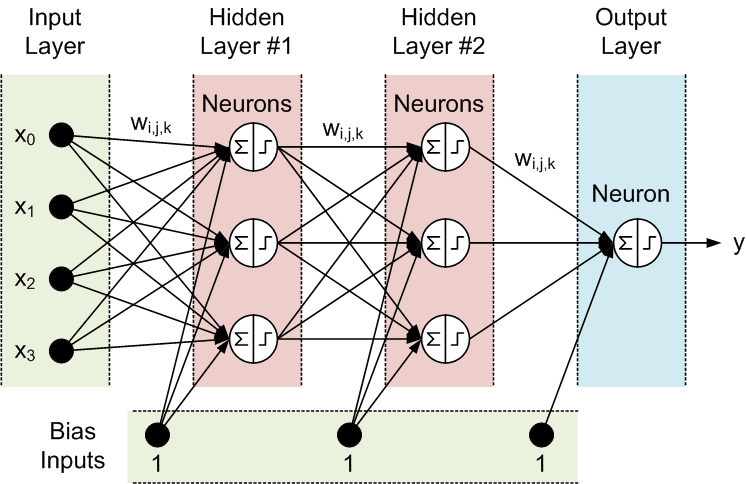The topology of a FFNN is often abbreviated as follows: <# of inputs> - <# of neurons in the first hidden layer> - <# of neurons in the second hidden layer> -...- <# of outputs>. The above network can be referred to as a 4-3-3-1 network.

The data is processed by neurons in two steps, correspondingly shown within the circle by a summation sign and a step sign:

1. All inputs are multiplied by the associated weights and summed
2. The resulting sums are processed by the neuron's activation function, whose output is the neuron output.

It is the neuron's activation function that gives nonlinearity to the neural network model. Without it, there is no reason to have hidden layers, and the neural network becomes a linear autoregressive (AR) model.

Enclosed library files for NN functions allow selection between three activation functions:

• sigmoid sigm(x)=1/(1+exp(-x)) (#0)
• hyperbolic tangent tanh(x)=(1-exp(-2x))/(1+exp(-2x)) (#1)
• rational function x/(1+|x|) (#2)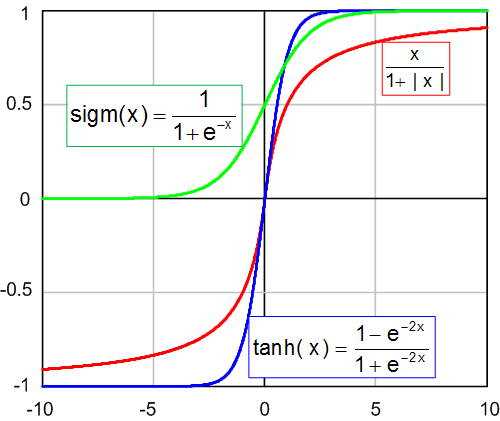The activation threshold of these functions is x=0. This threshold can be moved along the x axis thanks to an additional input of each neuron, called the bias input, which also has a weight assigned to it.

The number of inputs, outputs, hidden layers, neurons in these layers, and the values of the synapse weights completely describe a FFNN, i.e. the nonlinear model that it creates. In order to find weights the network must be trained. During a supervised training, several sets of past inputs and the corresponding expected outputs are fed to the network. The weights are optimized to achieve the smallest error between the network outputs and the expected outputs. The simplest method of weight optimization is the back-propagation of errors, which is a gradient descent method. The enclosed training function Train() uses a variant of this method, called Improved Resilient back-Propagation Plus (iRProp+). This method is described here

http://citeseerx.ist.psu.edu/viewdoc/summary?doi=10.1.1.17.1332

The main disadvantage of gradient-based optimization methods is that they often find a local minimum. For chaotic series such as a price series, the training error surface has a very complex shape with lots of local minima. For such series, a genetic algorithm is a preferred training method.

Enclosed files:

• BPNN.dll - library file
• BPNN.zip - archive of all files needed to compile BPNN.dll in C++
• BPNN Predictor.mq4 - indicator predicting future open prices
• BPNN Predictor with Smoothing.mq4 - indicator predicting smoothed open prices

File BPNN.cpp has two functions: Train() и Test(). Train() is used to train the network based on supplied past input and expected output values. Test() is used to compute the network outputs using optimized weights, found by Train().

Here is the list of input (green) и output (blue) parameters of Train():

double inpTrain[] - Input training data (1D array carrying 2D data, old first)
double outTarget[]
- Output target data for training (2D data as 1D array, oldest 1st)
double outTrain[]
- Output 1D array to hold net outputs from training
int ntr
- # of training sets
int UEW
- Use Ext. Weights for initialization (1=use extInitWt, 0=use rnd)
double extInitWt[]
- Input 1D array to hold 3D array of external initial weights
double trainedWt[]
- Output 1D array to hold 3D array of trained weights
int numLayers
- # of layers including input, hidden and output
int lSz[]
- # of neurons in layers. lSz is # of net inputs
int AFT
- Type of neuron activation function (0:sigm, 1:tanh, 2:x/(1+x))
int OAF
- 1 enables activation function for output layer; 0 disables
int nep
- Max # of training epochs
double maxMSE
- Max MSE; training stops once maxMSE is reached.

Here is the list of input (green) и output (blue) parameters of Test():

double inpTest[] - Input test data (2D data as 1D array, oldest first)
double outTest[]
- Output 1D array to hold net outputs from training (oldest first)
int ntt
- # of test sets
double extInitWt[]
- Input 1D array to hold 3D array of external initial weights
int numLayers
- # of layers including input, hidden and output
int lSz[]
- # of neurons in layers. lSz is # of net inputs
int AFT
- Type of neuron activation function (0:sigm, 1:tanh, 2:x/(1+x))
int OAF
- 1 enables activation function for output layer; 0 disables

Whether to use the activation function in the output layer or not (OAF parameter value) depends on the nature of outputs. If outputs are binary, which is often the case in classification problems, then the activation function should be used in the output layer (OAF=1). Please, pay attention that the activation function #0 (sigmoid) has 0 and 1 saturated levels whereas the activation functions #1 and 2 have -1 and 1 levels. If the network outputs is a price prediction, then no activation function is needed in the output layer (OAF=0).

### Examples of using the NN library:

BPNN Predictor.mq4 - predicts future open prices. The inputs of the network are relative price changes:

x[i]=Open[test_bar]/Open[test_bar+delay[i]]-1.0

where delay[i] is computed as a Fibonacci number (1,2,3,5,8,13,21..). The output of the network is the predicted relative change of the next price. The activation function is turned off in the output layer (OAF=0).

Indicator inputs:

extern int lastBar - Last bar in the past data
extern int futBars
- # of future bars to predict
extern int numLayers
- # of layers including input, hidden & output (2..6)
extern int numInputs
- # of inputs
extern int numNeurons1
- # of neurons in the first hidden or output layer
extern int numNeurons2
- # of neurons in the second hidden or output layer
extern int numNeurons3
- # of neurons in the third hidden or output layer
extern int numNeurons4
- # of neurons in the fourth hidden or output layer
extern int numNeurons5
- # of neurons in the fifth hidden or output layer
extern int ntr
- # of training sets
extern int nep
- Max # of epochs
extern int maxMSEpwr
- sets maxMSE=10^maxMSEpwr; training stops < maxMSE
extern int AFT
- Type of activ. function (0:sigm, 1:tanh, 2:x/(1+x))

The indicator plots three curves on the chart:

• red color - predictions of future prices
• black color - past training open prices, which were used as expected outputs for the network
• blue color - network outputs for training inputs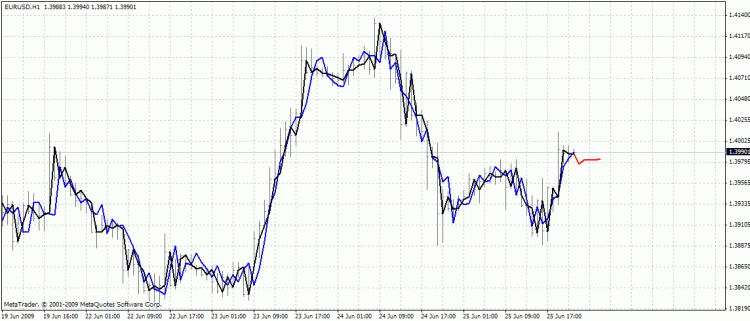BPNN Predictor.mq4 - predicts future smoothed open prices. It uses EMA smoothing with period smoothPer.

Setting all up:

1. Copy enclosed BPNN.DLL to C:\Program Files\MetaTrader 4\experts\libraries
2. In metatrader: Tools - Options - Expert Advisors - Allow DLL imports

You can also compile your own DLL file using source codes in BPNN.zip.

Recommendations:

• A network with three layers (numLayers=3: one input, one hidden and one output) is enough for a vast majority of cases. According to the Cybenko Theorem (1989), a network with one hidden layer is capable of approximating any continuous, multivariate function to any desired degree of accuracy; a network with two hidden layers is capable of approximating any discontinuous, multivariate function:
•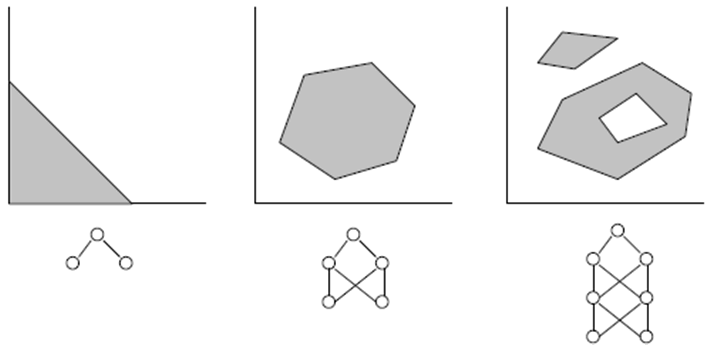• The optimum number of neurons in the hidden layer can be found through trial and error. The following "rules of thumb" can be found in the literature: # of hidden neurons = (# of inputs + # of outputs)/2, or SQRT(# of inputs * # of outputs). Keep track of the training error, reported by the indicator in the experts window of metatrader.
• For generalization, the number of training sets (ntr) should be chosen 2-5 times the total number of the weights in the network. For example, by default, BPNN Predictor.mq4 uses a 12-5-1 network. The total number of weights is (12+1)*5+6=71. Therefore, the number of training sets (ntr) should be at least 142. The concept of generalization and memorization (over-fitting) is explained on the graph below.
• The input data to the network should be transformed to stationary. Forex prices are not stationary. It is also recommended to normalize the inputs to -1..+1 range.

The graph below shows a linear function y=b*x (x-input, y-output) whose outputs are corrupted by noise. This added noise causes the function measured outputs (black dots) to deviate from a straight line. Function y=f(x) can be modeled by a feed forward neural network. The network with a large number of weights can be fitted to the measured data with zero error. Its behavior is shown as the red curve passing through all black dots. However, this red curve has nothing to do with the original linear function y=b*x (green). When this over-fitted network is used to predict future values of function y(x), it will result in large errors due to randomness of the added noise.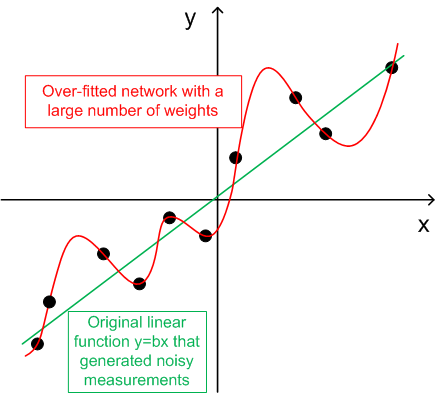In exchange for sharing these codes, the author has a small favor to ask. If you were able to make a profitable trading system based on these codes, please share your idea with me by sending email directly to vlad1004@yahoo.com.

Good luck!Fractal Dimension Index. + Step EMA

Linear Weighted Fractal Dimension Index (ternd vs notrend filter) plus Step EMABollinger Bands ® rev. by Jurik

Traditional Bollinger Bands ®, revisited with algorithm based on Jurik method.RFractals

Многоранговые фракталы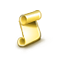A Tool: Horizontal Grid Plotter

Script for horizontal grid lines plotting